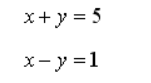# Solving equation with two unknowns

Hi this is my code for solving quadratic equation. This is working fine but problem is when I try to add one more unknown.

``````x = torch.nn.Parameter(torch.zeros(1), requires_grad=True)

def model(x):
b=3
a=2
c=1
global y
y = a*x ** 2 + b*x + c
return y

for i in range(150):
a = model(x)

loss = (0 - a) ** 2

loss.backward()
optimizer.step()
print(loss)
``````

I am computing loss from x. and my optimizer is optimizing x after every loop. When I change my equation to System of linear equations and I have one more unknown how could I calculate loss and update both unknowns ?
For example equation like thisHi Samuel!

Let’s say you have `nUnknowns` unknowns in your system and
also `nUnknowns` equations. Make the variable you optimize a
1d tensor of length `nUnknowns`:

``````x = torch.nn.Parameter (torch.zeros (nUnknowns))
``````

Then have your model return the left-hand sides of your `nUnknowns`
equations as a tensor of shape `[nUnknowns]` and supply the
right-hand sides of your equations as a `target` vector, also of shape
`[nUnknowns]`. You could calculate the loss “by hand” as you do in
your example, or you could use `MSELoss`:

``````loss = torch.nn.MSELoss() (model (x), target)
``````

(You could also have your model return a vector of differences
between the left-hand and right-hand sides of your equations,
and use its sum of squares as your loss.)

`torch.nn.Parameter` has `requires_grad = True)` by default,
so you don’t need to specify this explicitly. (But it doesn’t hurt to
do so.)

As a pure python comment, not specific to pytorch. in the context
of just the code you posted, `global y` doesn’t do anything. But, in
general, you should try to avoid `global`; there are usually cleaner,
“safer” ways of accomplishing whatever it is you might be trying to
do with `global`.

Best.

K. Frank

1 Like

To this sentence I want to ask you one more thing. “return a vector of differences” Please please, can you give me example how can I write this in python ? I understand what you mean but how to write vector like this in python. Thank you.

Hi Samuel!

Consider the following:

``````>>> import torch
>>> torch.__version__
'1.9.0'
>>>
>>> # your scalar x = z and y = z
>>> z = torch.nn.Parameter (torch.tensor ([3.0, 7.0]))   # just to make it more interesting than 0.0
>>> equationCoefficients = torch.tensor ([[1.0, 1.0], [1.0, -1.0]])
>>> rhs = torch.tensor ([5.0, 1.0])
>>> lhs = equationCoefficients @ z
>>> lhs